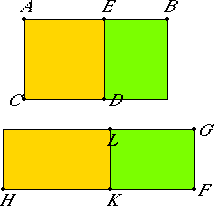# Proposition 108

If a medial area is subtracted from a rational area, then the side of the remaining area becomes one of two irrational straight lines, either an apotome or a minor straight line.

Let the medial area BD be subtracted from the rational area BC.

I say that the side of the remainder EC becomes one of two irrational straight lines, either an apotome or a minor straight line.

Set out a rational straight line FG, to FG apply the rectangular parallelogram GH equal to BC, and subtract GK equal to DB. Then the remainder EC equals LH.

Since, then, BC is rational, and BD medial, while BC equals GH, and BD equals GK, therefore GH is rational, and GK is medial.And they are applied to the rational straight line FG, therefore FH is rational and commensurable in length with FG, while FK is rational and incommensurable in length with FG. Therefore FH is incommensurable in length with FK.

X.73

Therefore FH and FK are rational straight lines commensurable in square only. Therefore KH is an apotome, and KF the annex to it.

Now the square on HF is greater than the square on FK by the square on a straight line either commensurable with HF or not commensurable.

First, let the square on it be greater by the square on a straight line commensurable with it.

X.Def.III.2

Now the whole HF is commensurable in length with the rational straight line FG set out, therefore KH is a first apotome.

X.91

But the side of the rectangle contained by a rational straight line and a first apotome is an apotome. Therefore the side of LH, that is, of EC, is an apotome.

X.Def.III.4

But, if the square on HF is greater than the square on FK by the square on a straight line incommensurable with HF, while the whole FH is commensurable in length with the rational straight line FG set out, then KH is a fourth apotome.

X.94

But the side of the rectangle contained by a rational straight line and a fourth apotome is minor.

Therefore, if a medial area is subtracted from a rational area, then the side of the remaining area becomes one of two irrational straight lines, either an apotome or a minor straight line.

Q.E.D.

## Guide

This proposition is not used in the rest of the Elements.